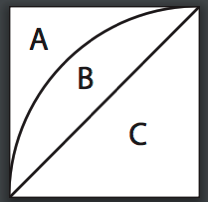Posted on

## Geometry 14.2 #22-23

Question from Joy:

We are in Geometry 3 rd edition. Chapter 14 lesson 2 number 22-23. Page 582.
How do you get 108?

The answer in key says 3 x 180/5?  Why? I think I missed something important yet simple. I was thinking divide 360/5. What an I doing wrong?  Thanks!

I have to admit – I could not find this in the book. I think it SHOULD be there – but could not find it.

The answer is the a triagle has interior angles that add up to 180 (we know that – right)

So each time we add a side (triangle to square) we add 180.

So in #22, he is saying we have 180 3 times (triangle plus 2 sides adding 2 more 180s) as the total angles and then dived by the number of sides to get 108.

Again – I do not see this in the book.

Hope this helps

dwc

Posted on

## Geometry Ch14 Test #4

Question: how do we work the problem on Chapter 14 test #4.

Since all 16 white regions are identical, we can find the area of one of them and multiply it by 16.

This figure represents one of the 16 small squares and one of the white regions.Since the diameter of the large circle in the figure shown on the test page is 4 units, the side of the square of one of the small squares is 1 unit.

We can find the area of region B by finding the area of regions B & C combined (a quarter of a circle) and subtracting the area of region C (a triangle):

> The area of B & C combined is1/4 (pi r squared) or 1/4 (pi 1 squared) = 1/4 pi

> The area of C is1/2 r squared or 1/2 1 squared = 1/2So B = (B + C) – C = 1/4 pi – 1/2 and the total area of the 16 white regions is16(1/4 pi – 1/2) = 4 pi – 8.

Posted on

## Geometry Ch14.3 #34

Question from Anna:

Jacob’s Geometry Chapter 14 Lesson 3 Problem #34. How do you work out this problem?

I wrote this one out on graph paper for you. Click on the graphic to make it larger if needed. Hope it helps!

Posted on

## Geometry Ch14.2 #22 #23

Question from John:

Please explain the answer given in the Enhanced Teacher’s Guide for Chapter 14, Lesson 2, Number 22, 23.

The method implied in Jacob’s Enhanced Teacher’s Guide is based on dividing the polygon into triangles by drawing diagonals from one vertex. This is illustrated by exercises #38-51 of Chapter 7, Lesson 1 (pages 262-263).

Posted on

## Geometry Ch14.3 #16, #17, #34

Question from Jackson:

I am on chapter 14 in geometry learning about the areas of regular polygons.  I am having trouble understanding how they got the answers. Ex: 14.3 #16-19 I don’t know how to relate r to a. Are they talking in terms of the area of the square? I saw the answer and I have no Idea how they got to a square root.
Also, later in the lesson #34 and the others talking about relations, I just can’t grasp the concepts of relating the terms.

For #17: Based on the other problems, you should see that this is a right triangle and PB = a. So both sides the same, or a 45-45-90 degree triangle.

So by pythagorean theorem

a^2 + b^2 = c^2

but in this case it is a^2 + a^2 = r^2

so we have 2a^2 = r^2

For number 16, now to get in terms of r, just solve for r by taking square root.

for 34, based on the two problems above, you know area of a triangle is 1/2 of its height times its base. But since you have two triangle of same area in the WHOLE triangle, you now have area of AOB

= a * r/2

and r/2 was equal to that in last problem – so multiply out.